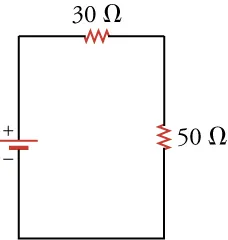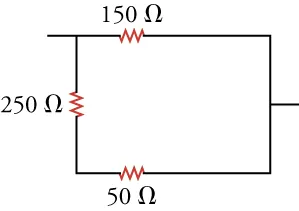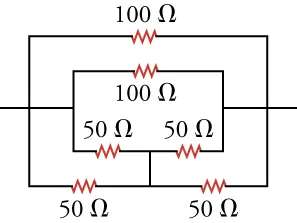Physics

# Problems

PhysicsProblems

### Problems

#### 19.1Ohm's law

20.

What voltage is needed to make 6 C of charge traverse a 100-Ω resistor in 1 min?

1. The required voltage is 1 × 10−3 V.
2. The required voltage is 10 V.
3. The required voltage is 1,000 V.
4. The required voltage is 10,000 V.
21.

Resistors typically obey Ohm’s law at low currents, but show deviations at higher currents because of heating. Suppose you were to conduct an experiment measuring the voltage, V, across a resistor as a function of current, I, including currents whose deviations from Ohm’s law start to become apparent. For a data plot of V versus I, which of the following functions would be best to fit the data? Assume that a, b, and c are nonzero constants adjusted to fit the data.

1. $V=aI V=aI$
2. $V=aI+b V=aI+b$
3. $V=aI+b I 2 V=aI+b I 2$
4. $V=aI+b I 2 +c V=aI+b I 2 +c$
22 .
A battery of unknown voltage $V_1$ is attached across a resistor $R_1$. You add a second battery with $V_2 = 9.0\,\text{V}$ in series with $V_1$ so that the voltage across $R_1$ is now $V_1 + V_2$ and measure $0.3\,\text{A}$ of current through resistor $R_1$. You add a third battery with $V_3 = 9.0\,\text{V}$ in series with the first two batteries so that the voltage across $R_1$ is $V_1 + V_2 + V_3$ and measure $0.4\,\text{A}$ of current through $R_1$. What is the resistance of $R_1$?
1. $23.25\,\Omega$
2. $21.75\,\Omega$
3. $31.33\,\Omega$
4. $90\,\Omega$

#### 19.2Series Circuits

23.

What is the voltage drop across two 80-Ω resistors connected in series with 0.15 A flowing through them?

1. 12 V
2. 24 V
3. 36 V
4. 48 V
24.

In this circuit, the voltage drop across the upper resistor is 4.5 V. What is the battery voltage?1. 4.5V
2. 7.5V
3. 12V
4. 18V

#### 19.3Parallel Circuits

25.

What is the equivalent resistance of this circuit?1. The equivalent resistance of the circuit is 32.7 Ω.
2. The equivalent resistance of the circuit is 100 Ω.
3. The equivalent resistance of the circuit is 327 Ω.
4. The equivalent resistance of the circuit is 450 Ω.
26.

What is the equivalent resistance of the circuit shown below?1. The equivalent resistance is 25 Ω.
2. The equivalent resistance is 50 Ω.
3. The equivalent resistance is 75 Ω.
4. The equivalent resistance is 100 Ω.

#### 19.4Electric Power

27.

When 12 V are applied across a resistor, it dissipates 120 W of power. What is the current through the resistor?

1. The current is 1,440 A.
2. The current is 10 A.
3. The current is 0.1 A.
4. The current is 0.01 A.
28.

Warming 1 g of water requires 1 J of energy per . How long would it take to warm 1 L of water from 20 to 40 °C if you immerse in the water a 1-kW resistor connected across a 9.0-V batteries aligned in series?

1. 10 min
2. 20 min
3. 30 min
4. 40 min
Order a print copy

As an Amazon Associate we earn from qualifying purchases.